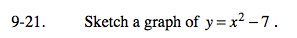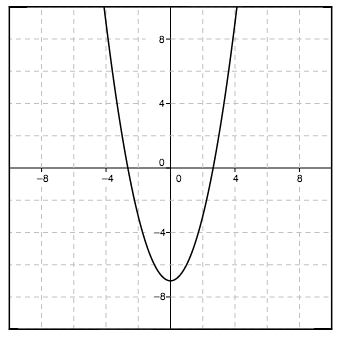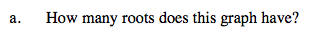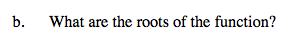Home > A2C > Chapter 9 > Lesson 9.1.1 > Problem9-21

9-21.
1. Sketch a graph of y = x2 − 7. Homework Help ✎

1. How many roots does this graph have?

2. What are the roots of the function?How many times does the graph intersect the x-axis?